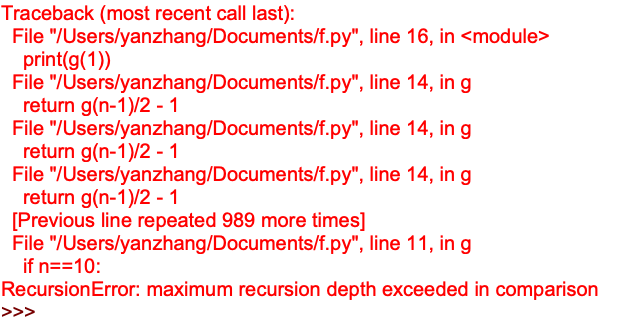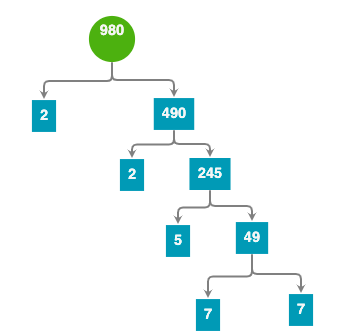# 轻松搞懂Python递归函数的原理与应用

2019/04/10 10:10

1、递归的原理## 2、递归的玩法

### (1) 认清自己

f(n) = (f(n-1) + 1)*2 ，告诉你f(1)=1，问f(10)是多少？

def f(n):
if n==1:
return 1
else:
return (f(n-1)+1)*2

print(f(10))

### （2）把握好方向

g(n) = g(n-1)/2 -1 ，告诉你 g(10)=1，问g(1) 是多少？

def g(n):
if n==10:
return 1
else:
return g(n-1)/2 - 1

print(g(1))

运行后却出现了如下的错误，提示递归溢出！这是怎么回事呢？因为我们还有第2个点，您没有注意！

-2.0

g(n-1) = (g(n)+1) * 2    => g(n) = (g(n+1)+1) * 2

1 def g(n):
2     if n==10:
3         return 1
4     else:
5         return 2*(g(n+1)+1)
6
7 print(g(1))

## 3、分解质因数练习

1 def defactor(N):  #定义一个函数名称为defactor，意义是返回N的所有因子
2     for i in range(2,N): #从2开始试试
3         if N%i ==0: #如果试到i是N的因子的话，就返回i的所有因子和N/i的所有因子 的列表
4             return defactor(i)+defactor(int(N/i))
5     else:#如果没有试到就说明这个N是一个质数，就直接包含它的 列表
6         return [N]
7
8 print(defactor(980))

[2, 2, 5, 7, 7]

Bingo，在这里函数defactor(N

（1）定义:  求出参数N所有因子的数组，

（2）方向：整数 -> 最小的质数0
0 收藏

### 作者的其它热门文章0 评论
0 收藏
0